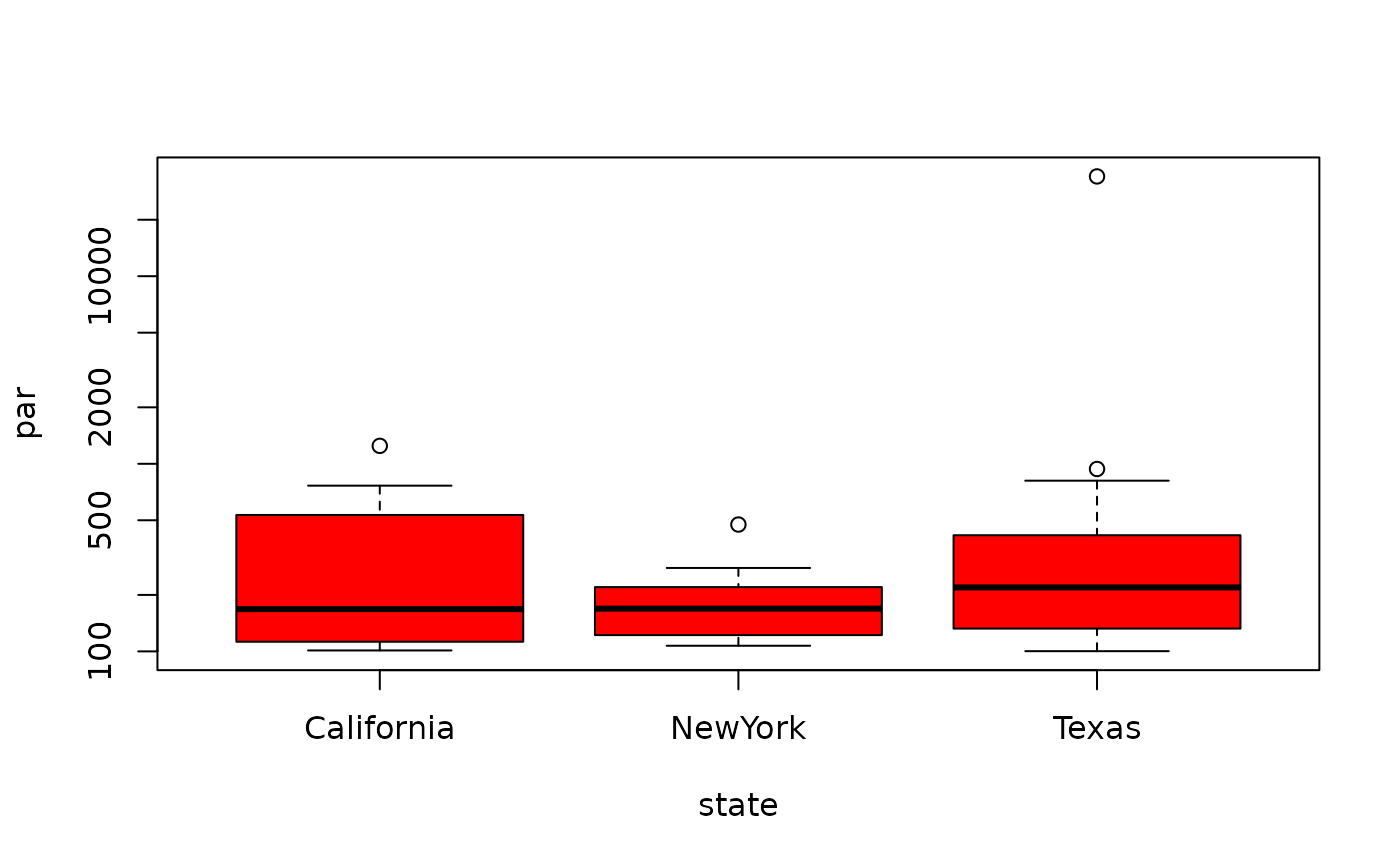Data for Exercise 10.34 and 10.49

Saving

## Format

A data frame/tibble with 65 observations on two variables

par

problem-asset-ratio for Savings & Loans that were listed as being financially troubled in 1992

state

U.S. state

## Examples


boxplot(par ~ state, data = Saving, col = "red")boxplot(par ~ state, data = Saving, log = "y", col = "red")model <- aov(par ~ state, data = Saving)
summary(model)
#>             Df    Sum Sq  Mean Sq F value Pr(>F)
#> state        2 2.269e+07 11345362   0.638  0.532
#> Residuals   62 1.103e+09 17784535
plot(TukeyHSD(model))kruskal.test(par ~ factor(state), data = Saving)
#>
#> 	Kruskal-Wallis rank sum test
#>
#> data:  par by factor(state)
#> Kruskal-Wallis chi-squared = 1.708, df = 2, p-value = 0.4257
#>